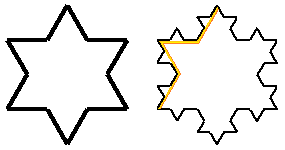BFOIT - Introduction to Computer Programming

# Von Koch Snowflake Program Hint #2

Still stuck?

Here is where we stand.  We have the following procedures

 `````` ; Draw a Von Koch Curve ; level - count of number of times to break a straight line ; into three parts: left side, middle, and right ; side. When equal to zero, simply draw a straight ; line. ; steps - length of the curve/line drawn in turtle steps to kochCurve :level :steps if equal? :level 0 [forward :steps stop]    forward (quotient :steps 3) left 60 forward (quotient :steps 3) right 120 forward (quotient :steps 3) left 60 forward (quotient :steps 3) end to kochFlake :level :steps setheading 30 repeat 3 [kochCurve :level :steps right 120]    end``````

Let's take a step back.  If we extract the code that draws the level 1 kochCurve into its own procedure, kochCurve1, and fix kochCurve to invoke it, we have the following.

 `````` to kochCurve1 :steps forward (quotient :steps 3) left 60 forward (quotient :steps 3) right 120 forward (quotient :steps 3) left 60 forward (quotient :steps 3) end to kochCurve :level :steps if equal? :level 0 [forward :steps stop]    if equal? :level 1 [kochCurve1 stop]    end``````

Now let's write kochCurve2.  Just to refresh you memory, here are Koch snowflakes for level 1 and level 2.  I've also annotated the level 2 snowflake with one kochCurve from level 1.For level 2, we need to replace what were straight lines in level 1 with what look like kochCurve1s.  So here is where we stand with a new kochCurve2 and a modified kochCurve.

 `````` to kochCurve :level :steps if equal? :level 0 [forward :steps stop]    if equal? :level 1 [kochCurve1 stop] if equal? :level 2 [kochCurve2 stop] end to kochCurve1 :steps forward (quotient :steps 3) left 60 forward (quotient :steps 3) right 120 forward (quotient :steps 3) left 60 forward (quotient :steps 3) end to kochCurve2 :steps kochCurve1 (quotient :steps 3)    left 60 kochCurve1 (quotient :steps 3) right 120 kochCurve1 (quotient :steps 3) left 60 kochCurve1 (quotient :steps 3) end``````

So writing kochCurve2 wasn't that difficult.  Just as the figure above shows, it simply invokes kochCurve1 in place of the forward command.  We are looking for a pattern that has the appearance of a recursive process and we are on to something here.

Is writing a kochCurve3 procedure just as simple?  Do we just copy/paste the definition of kochCurver2, change its name and replace kochCurve1 invocations in it with invocations of kochCurve2?  If so, then adding another if instruction to kochCurve would get us a level 3 snowflake!

Try it out...  Here is a modified kochCurve and a new kochCurve3.

 `````` to kochCurve :level :steps if equal? :level 0 [forward :steps stop]    if equal? :level 1 [kochCurve1 stop] if equal? :level 2 [kochCurve2 stop] if equal? :level 3 [kochCurve3 stop] end to kochCurve3 :steps kochCurve2 (quotient :steps 3)    left 60 kochCurve2 (quotient :steps 3) right 120 kochCurve2 (quotient :steps 3) left 60 kochCurve2 (quotient :steps 3) end``````

It works... doesn't it?  Well, we have a pattern begging for a recursive solution.

We discovered the pattern by...

There is still one exception to the pattern we've uncovered, kochCurve1 does not invoke kochCurve.  It is using forward to draw a straight line.  If you think about it, we could remove the forward commands from kochCurve1.  We know that if we invoke kochCurve with its first input (level) equal to zero, it does the same thing as forward.  Here we go.

 `````` to kochCurve1 :steps kochCurve 0 (quotient :steps 3)    left 60 kochCurve 0 (quotient :steps 3) right 120 kochCurve 0 (quotient :steps 3) left 60 kochCurve 0 (quotient :steps 3) end``````

We are still looking for a way to simplify our program with recursion.  But now you should see the emergence of a pattern.

Can you figure out how to eliminate kochCurve1 and kochCurve2, and kochCurve3, leaving only kochCurve?  Remember... kochCurve1 came out of kochCurve.This work (BFOIT: Introduction to Computer Programming, by Guy M. Haas),
identified by Berkeley Foundation for Opportunities in IT (BFOIT),
is free of known copyright restrictions.